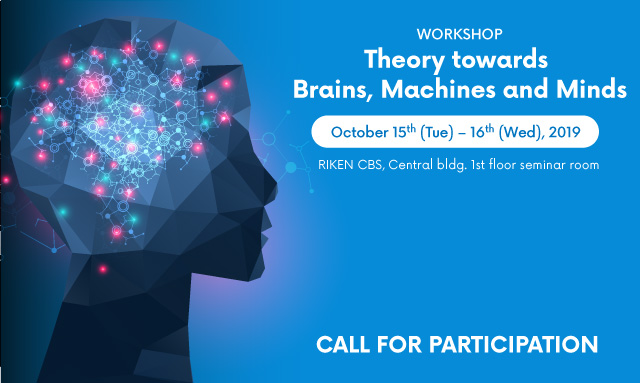## Title: Machine learning for high-dimensional big data

Kei Majima, Graduate School of Informatics, Kyoto University

Statistical machine learning methods are increasingly being used to reveal information encoded in measured neural data. With advances of measurement technology in the recent decades, data with a larger number of dimensions are treated, and effective methods of analyzing such high dimensional data have been extensively developed. In this talk, I will focus on two types of problems that arise in high dimensional data analysis, overfitting and large computational cost. In the first half, I will explain that sparse estimation efficiently prevents overfitting and is useful in constructing a better neural decoding model while introducing our recently developed method, sparse ordinal logistic regression (Satake et al., 2018) as an example. In the second half, I will show several approaches for reducing the computational cost when we treat data with a huge number of dimensions. By taking principal component analysis and canonical correlation analysis as examples, I will explain quantum-inspired algorithms, recently developed randomized algorithms for linear algebra computations, provide manners to exponentially speed up these data analyses (Koide-Majima & Majima, 2019). Then, I will discuss advantages, disadvantages, and possible applications of these recently developed algorithms.

References:
 Koide-Majima N., Majima K., “Quantum-inspired canonical correlation analysis for exponentially large dimensional data,” arXiv preprint, 2019.
 Satake E., Majima K., Aoki S.C., Kamitani Y., “Sparse ordinal logistic regression and its application to brain decoding,” Frontiers in Neuroinformatics, Vol. 12, 51, 2018.